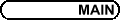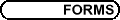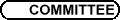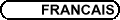Harmonic Analysis / Analyse harmonique
Org: Galia Dafni (Concordia)

JAMES COLLIANDER, University of Toronto, 100 St. George Street, Toronto, ON M5S 3G3
On rough blowup solutions of L2 critical NLS

The goal of the work reported upon in this talk is to understand the L2 critical nonlinear Schrödinger (NLS) blowup dynamics for rough initial data. Much of the known theory for NLS blowup relies upon energy conservation and is thus restricted to H1 solutions, even though the NLS evolution problem is well-posed in L2. Two new results will be described:

(1) For all s satisfying sQ < s < 1, Hs blowup solutions concentrate at least the mass of the ground state soliton into a point at blowup time.
(2) L2 blowup solutions concentrate at least a fixed amount (conjectured to be the mass of the ground state) of L2 mass at blowup time.

PAUL GAUTHIER, Université de Montréal, Centreville, Montréal, QC H3C 3J7
Completeness of certain function spaces

We recall the maximum principle on unbounded domains and discuss some recent applications thereof to determine when certain spaces of harmonic and holomorphic functions are complete.

PENGFEI GUAN, McGill University
Homogeneous Weingarten curvature equations

If M is a starshaped hypersurface about the origin in Rn+1, by dilation property of the curvature function, the k-th Weingarten curvature can be considered as a function of homogeneous degree -k in Rn+1 \{0}. The homogeneous Weingarten curvature problem is: given a homogeneous function F of degree -k in Rn+1 \{0}, does there exist a starshaped hypersurface M such that its k-th Weingarten curvature is at x Î M is equal to F(x)? The problem is a nonlinear eigenvalue problem for the curvature equation. When k=n, the resultant equation is a Monge-Ampère type, the problem was studied by Delanoë; on the other end k=1, the equation is of mean curvature type which was studied by Treibergs. We will describe a recent joint work with X. Ma and C. S. Lin on the problem for all cases 1 £ k £ n. We also discuss the existence of convex solutions.

KATHRYN HARE, Department of Pure Mathematics, University of Waterloo, Waterloo, ON N2L 3G1
Energy and Hausdorff dimensions of measures

Energy and Hausdorff dimension are two related ways to quantify the singularity of a measure. There is a classical formula relating the energy of a positive measure on Rn with a weighted L2 norm of its Fourier transform. We discuss an extension of this formula to measures on compact, connected, Riemannian manifolds, replacing the Fourier transform with the projections of the measures onto the eigenspaces of the Laplacian operator. We also discuss extending the definition of energy to signed measures and show that this generalized energy dimension continues to provide a lower bound for the Hausdorff dimension of the measure.

XINHOU HUA, University of Ottawa
Meromorphic Functions Sharing the Same Zeros and Poles

In this paper, we solved Hinkkanen's problem proposed in 1984: Any meromorphic function f is determined by its zeros and poles and the zeros of its first four derivatives. We also show that the number 4 is best possible by a counterexample.

MARIANNE KORTEN, Kansas State University, Department of Mathematics, 138 Cardwell Hall, Manhattan, KS 66502
Existence, uniqueness and regularity of the free boundary in the Hele-Shaw problem

We obtain the unique weak solution (u¥,V) to the Hele-Shaw problem with a mushy zone 0 £ u¥ £ 1

 u¥t = DV(x,t) Î Gc ×(0,+¥),
 u¥ (x,0) = uI (x),
 V(x,t) = p(x),     (x,t) Î ¶G ×(0,+¥).
(1)

This model describes the flow of a viscous fluid being injected through the slot G between two nearby plates, and is used in injection molding for the production of packaging materials and the interior plastic parts of cars and airplanes, in electromechanical machining, and to study the diffusion of nutrients and medicines within certain tumors.

We find u¥, and V as the (pointwise) "Mesa" type limit of u(m) and u ( um, m(um-1)+ ), where the um are solutions to one-phase Stefan problems with increasing diffusivities u(m)t = D(u(m)-1)+, with fixed initial and boundary data 0 £ uI £ 1 and p(x) > 0. The main tools are information available from Korten's earlier work about solutions and interphases in the one-phase Stefan problem, Blank's results on the obstacle problem, and new energy estimates for Stefan problems due to Moore and Korten.

We obtain the traditional formulation (by means of the Baiocchi transformation) as an obstacle problem. At this point regularity in space follows from the work of Blank and Caffarelli.

Joint work with I. Blank and C. N. Moore.

ISABELLA LABA, University of British Columbia, Vancouver, BC
Wolff's inequality for hypersurfaces

Wolff (2000) proved an Lp "local smoothing" inequality for circular cones in dimension 3 and for large enough p, and used it to obtain sharp Lp estimates for cone multipliers and local smoothing bounds for solutions of the wave equation. This result was extended to higher dimensions by Wolff and myself. In a joint work with Malabika Pramanik, we extend it further to a wider class of hypersurfaces of codimension 1, including generic surfaces with negative curvatures and certain surfaces with more than one flat direction.

AKOS MAGYAR, University of Georgia
A note on Fourier restriction and the Newton polygon

Local Lp - L2 bounds are proved for the restriction of the Fourier transform to analytic surfaces of the form: S = ( x,f(x) ) in R3. It is found that the range of exponents are determined by the so-called distance of the Newton polygon, associated to f, except when the principal part of f(x) contains a factor of high multiplicity. The proofs are based on the method of Phong-Stein and Rychkov, adapted to scalar oscillatory integrals.

One Multiplier theorem, several proofs

A model subspace of the Hardy space H2 generated by the inner function Q is KQ = H2 - Q H2. The model subspaces generated by Q = ei sQ, s > 0 have been extensively studied. The classical mutiplier theorem of Beurling-Malliavin characterizes the admissible majorants of these special and very important model subspaces of H2. The original proof is rather difficult. Thereafter, many mathematicians, including in particular Paul Koosis, worked in this direction and some gave new proofs of the classical theorem. Victor Havin, Fedya Nazarov and I also gave a new proof. We believe that our proof is the simplest one and besides our main theorem also works for other model subspaces of H2.

MING MEI, Concordia University, Montreal, Canada
Phase transitions to 2×2 system of conservation laws with periodic boundary condition

The study focuses on the phase transitions of a 2×2 p-system of viscosity-capillarity with periodic initial-boundary condition in viscoelastic material. The goal of the present study is to make a novel contribution to the open problem: the asymptotic behavior of the solution of this model. The location of the initial data and the amplitude of viscosity play a key role for the phase transitions. The criteria of the phase transition solutions are provided for both the original problem and its steady-state periodic boundary value problem. Furthermore, the convergence rate of the steady-state solutions is obtained. A crucial step in this study is the proof of the uniform boundedness of the solution by Liapunov functional. Finally, numerical simulations are carried out to confirm the theoretical results.

This is a joint work with Yau Shu Wong at University of Alberta and Liping Liu at Duke University.

MARIUS MITREA, University of Missouri
The solution of the Chang-Krantz-Stein conjecture

In this talk I will report on some recent joint work with S. Mayboroda on optimal regularity results for the harmonic Green potential G on Besov and Triebel-Lizorkin scales in Lipschitz domains. As is well-known, the latter class contains, as a particular case, the Hardy space Hp. When specialized to this setting, our results prove that two derivatives on G map Hp to itself for a small interval of values p < 1. For Lipschitz domains, this has been conjectured to be the case by Chang-Krantz-Stein in the early 1990s.

CAMIL MUSCALU, Cornell University
Paraproducts on polydiscs

Last year, at the AMS/CMS Meeting in Vancouver, we presented a result which extended the classical Coifman-Meyer theorem to the bi-parameter setting of the bidisc. We also pointed out that the d-parameter case is open, for d > 2. This open case has been resolved in the meantime and the corresponding theorem will be described during our talk.

This is recent joint work with Jill Pipher, Terry Tao and Christoph Thiele.

PHILIPPE POULIN, McGill University, 845 rue Sherbrooke Ouest, Montreal, H3A 2T5
The Molchanov-Vainberg Laplacian

It is well known that the Green function of the standard discrete Laplacian on a lattice exhibits a pathological behavior in dimension greater than 2. Molchanov and Vainberg suggested an alternative to the usual Laplacian and conjectured that a polynomial decay holds for this latter. This talk presents a proof of this conjecture.

TOM RANSFORD, Université Laval, Dép. de mathématiques, Québec (QC), G1K 7P4
Invariant subspaces of the Dirichlet space

One of the cornerstones of the theory of Hardy spaces is Beurling's invariant-subspace theorem, which classifies the closed subspaces invariant under the shift operator. The corresponding classification in the Dirichlet space is still an open problem. I will discuss some recent progress in this area.

Joint work with Omar El-Fallah and Karim Kellay.

ERIC SAWYER, McMaster University, Hamilton, Ontario
Besov spaces on complex balls: interpolating sequences and trees

The theory of interpolating sequences has its roots in the work of Lennart Carleson on the corona problem. We characterize interpolating sequences for Besov spaces on complex balls in dimension greater than one, extending the recent one dimensional work of B. Boe, using a new concept of holomorphic tree.

This is joint work with Nicola Arcozzi and Richard Rochberg.

ALINA STANCU, Université de Montréal & Polytechnic University of NY
Some uniqueness results to a class of differential equations

Let g be a positive 2p-periodic function and let p be some real number. Assuming solutions to the equation u1-p (u"+u) = g exist, we will discuss the use of curvature flows and convex geometry in counting the number of solutions.

PAUL TAYLOR, McMaster University, 1280 Main W, Hamilton, ON L8S 4K1
Bochner-Riesz Means With Respect to a Rough Distance Function

The generalized Bochner-Riesz operator may be defined as as a Fourier multiplier operator, where the multiplier is given by taking the distance to a surface and raising this distance to a positive power. The behaviour of the operator is described when the surface is taken to be a cylinder, which results in a rough distance function.

DIMITER VASSILEV, CRM/ISM and UQAM
Analytic continuation of the functions Pl

Let P be a function defined and continuous near the origin of RN. Consider the integral

 JP (l) = óõ Br |P(x)|l  dV,
(1)
where dV is the Lebesgue measure on RN. For a fixed P the above integral converges absolutely when the real part of l is positive. Furthermore, it defines a holomorphic function of l in this region. A natural question is to find spaces of functions for which JP (l) has a holomorphic extension to the left of the imaginary axis for any P in the considered space. One can also ask if the extension is uniform with respect to "small" perturbations of P.

We shall present an approach to the above questions for the space of local solutions to an elliptic equation with Lipschitz coefficients.

JIE XIAO, Memorial University of Newfoundland, St. John's, NL A1C 5S7
The Heat Equation I: Carleson-Sobolev Estimates

We give Carleson type estimates for an operator valued solution of the heat equation with initial data in the homogeneous Sobolev space.